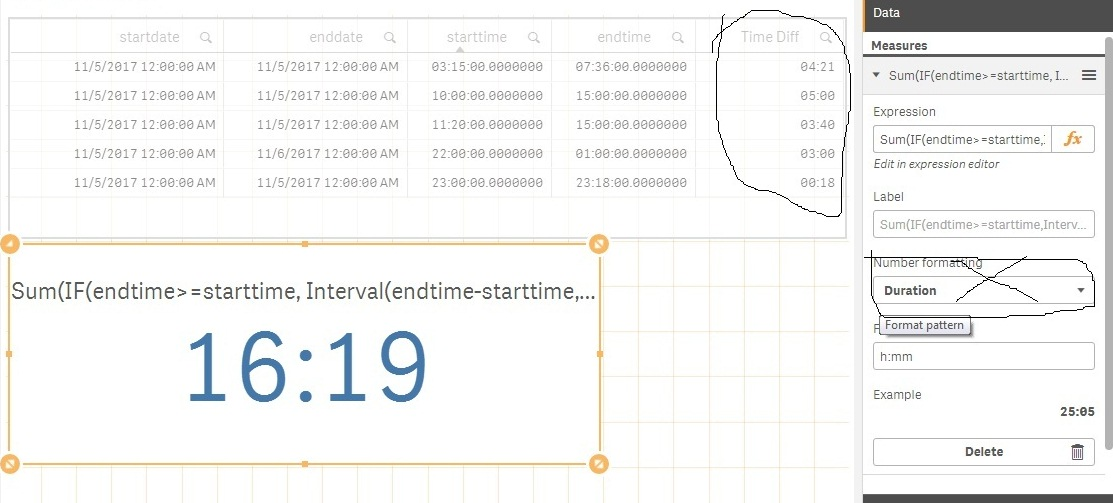# New to Qlik Sense

Discussion board where members can get started with Qlik Sense.

Announcements
Qlik® Product Spotlight: Discover what’s possible. Get more from our products.
See for yourself. Register today.
Contributor II

## How to calculate sum of Time

Hi Everyone

I want to calculate Sum of Time Diff in KPI without using 'Duration' property. Please view snapshot.

I am using this expression:

Sum(IF(endtime>=starttime,

Interval(endtime-starttime,'hh:mm'),TIME(Interval#('24:00','hh:mm')-Interval(starttime-endtime,'hh:mm'),'hh:mm')

))

If I use number formatting as Auto it gives 0.68 i.e. wrong.

I want the result without using Duration. I want the solution using Expression(Formula).1 Solution

Accepted SolutionsMVP

## Re: How to calculate sum of Time

May be this

Interval(Sum(If(endtime >= starttime, endtime-starttime, MakeTime(24)-starttime-endtime)), 'hh:mm')

5 Replies
Esteemed Contributor

## Re: How to calculate sum of Time

hi,

on the screen shot, the TIME DIFF are correct ? if so ? how much would be the correct sum of time ?

Esteemed Contributor

## Re: How to calculate sum of Time

If you want to keep it on auto; you'll have to directly format your expression :

time#[Sum(IF(endtime>=starttime,

Interval(endtime-starttime,'hh:mm'),TIME(Interval#('24:00','hh:mm')-Interval(starttime-endtime,'hh:mm'),'hh:mm')

)),'hh:mm')

Contributor II

## Re: How to calculate sum of Time

Correct SUm of time is 16:19 but it is coming when I use Duration Formating. I want to do with expressionMVP

## Re: How to calculate sum of Time

May be this

Interval(Sum(If(endtime >= starttime, endtime-starttime, MakeTime(24)-starttime-endtime)), 'hh:mm')

Contributor II

Thanks Sunny